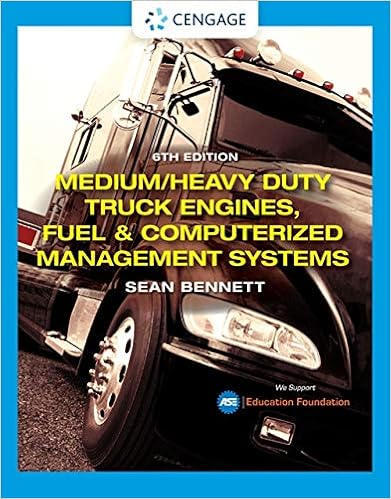Of the fluid in the stagnation tube 5 the water level

• Homework Help
• 3

This preview shows page 1 - 3 out of 3 pages.

We have textbook solutions for you!
The document you are viewing contains questions related to this textbook.The document you are viewing contains questions related to this textbook.
Chapter 3 / Exercise 3
Medium/Heavy Duty Truck Engines, Fuel & Computerized Management Systems
BennettExpert Verified
of the fluid in the stagnation tube. 5. The water level in a tank is 20 m above the ground. A hose is connected to the bottom of the tank, and the nozzle at the end of the hose is pointed straight up. The tank cover is airtight, and the air pressure above the water surface is 1 atm gage. The system is at sea level. Determine the maximum height to which the water stream could rise. (1 atm = 101.325 kPa).
We have textbook solutions for you!
The document you are viewing contains questions related to this textbook.The document you are viewing contains questions related to this textbook.
Chapter 3 / Exercise 3
Medium/Heavy Duty Truck Engines, Fuel & Computerized Management Systems
BennettExpert Verified
ENGR 2097 Assignment #5 Solutions Q1: According to the mass conservation, the volumetric flow rate into the tank for this imcompressible liquid is ) 4 / )( ( ) 4 / ( ) 4 / ( 2 2 2 d V V d V d V V V V out in out in outlet inlet filling π π π - = - = - = & & & The volume to fill is ) 3 . 0 1 )( ( 2 m m D V empty - = π The required time: 45.57s = - = - - = = 2 2 2 2 ) 12 . 0 )( / 9 . 1 / 5 . 2 ( ) 7 . 0 ( ) 75 . 0 ( ) 4 / )( ( ) 3 . 0 1 )( 4 / ( m s m s m m m d V V m m D V V t out in filling empty π π & Q2: Mass conservation between the inlet and outlet: [ ] [ ] 2 max 7 / 15 7 / 15 7 / 1 max 0 7 / 15 7 / 8 7 / 1 max 0 7 / 8 7 / 1 7 / 1 max 0 7 / 1 7 / 1 max 0 7 / 1 7 / 1 max 2 0 0 7 / 1 max 0 0 0 2 1 2 1 2 1 60 49 ) 15 / 7 ( ) 8 / 7 ( ) / 2 ( ) 15 / 7 ( ) 8 / 7 ( ) / 2 ( ) ( ) / 2 ( ) )( ( ) ( ) / 2 ( ) with (replacing ) ( ) / 2 ( ) ( ) 2 ( 1 ) 2 )( ( ) 2 ( , , R U R R R U x Rx R U dx x Rx R U dx x R x R U x R-r rdr r R R U R U dr r R r U dr r r u dr r U V V V V m m R R R R R R R π π π π π π π π π π ρ ρ = - = - = - = - - = - = - = = = = = & & & & 60 49 max = U U o Q3: As the duct converges to a smaller cross-sectional area, the velocity increases. By Bernoulli’s equation, the pressure therefore decreases. Thus Manometer A
•••